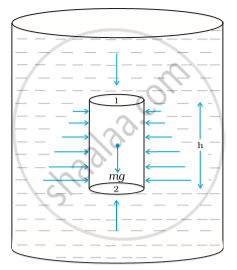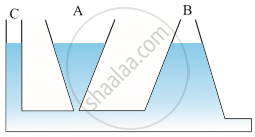# Pressure - Variation of Pressure with Depth

#### notes

Variation of pressure with depth

• Consider a cylindrical object inside a fluid; consider 2 different positions for this object.

• The fluid is at rest therefore the force along the horizontal direction is 0.

• Forces along the vertical direction:-

• Consider two positions 1 and 2.

• Force at position 1 is perpendicular to cross-sectional area A, F1= P1

• Similarly F2=P2

• Total force Fnet= -F1+F2 as F1 is along negative y-axis therefore it is –ive. And F2 is along the +ive y-axis.

• Fnet = (P2-P1)A
• This net force will be balanced by the weight of the cylinder(m).

Therefore under equilibrium condition:

Fnet=mg=weight of the cylinder = weight of the fluid displaced.

=ρVg where ρ=density=volume of the fluid

=ρhAg where V=hA(h=height and A= area)

Therefore (P2-P1) A=ρhAg

thereforeP2-P1 = ρhg

Therefore the difference in the pressure is dependent on the height of the cylinder.

Consider the top of the cylinder exposed to air therefore P1=Pa(where Pa= P1 is equal to atmospheric pressure.)

Then P2=Pa+ ρhg

Conclusion: The pressure P, at depth below the surface of a liquid open to the atmosphere is greater than atmospheric pressure by an amount ρhg.

The pressure is independent of the cross-sectional or base area or the shape of the container.• Consider 3 vessels of very different shapes (like thin rectangular shape, triangular and some filter shape) and we have a source from which water enters into these 3 vessels.

• Water enters through the horizontal base which is the base of these 3 vessels we observe that the level of water in all the 3 vessels is the same irrespective of their different shapes.

• This is because the pressure at some point at the base of these 3 vessels is the same.

• The water will rise in all these 3 vessels till the pressure at the top is the same as the pressure at the bottom.

• As pressure is dependent only on height therefore in all the 3 vessels the height reached by the water is the same irrespective of the difference in their shapes.

• This experiment is known as Hydrostatic Paradox.If you would like to contribute notes or other learning material, please submit them using the button below.